# Recipes

Various recipes implemented in Auto-FOX.

 FOX.recipes.param A set of functions for analyzing and plotting ARMC results. FOX.recipes.psf A set of functions for creating .psf files. FOX.recipes.ligands A set of functions for analyzing ligands. FOX.recipes.time_resolution A set of functions for calculating time-resolved distribution functions. FOX.recipes.similarity Recipes for computing the similarity between trajectories.

## FOX.recipes.param

A set of functions for analyzing and plotting ARMC results.

Examples

A general overview of the functions within this module.

>>> import pandas as pd
>>> from FOX.recipes import get_best, overlay_descriptor, plot_descriptor

>>> hdf5_file: str = ...

>>> param: pd.Series = get_best(hdf5_file, name='param')  # Extract the best parameters
>>> rdf: pd.DataFrame = get_best(hdf5_file, name='rdf')  # Extract the matching RDF

# Compare the RDF to its reference RDF and plot
>>> rdf_dict = overlay_descriptor(hdf5_file, name='rdf')
>>> plot_descriptor(rdf_dict)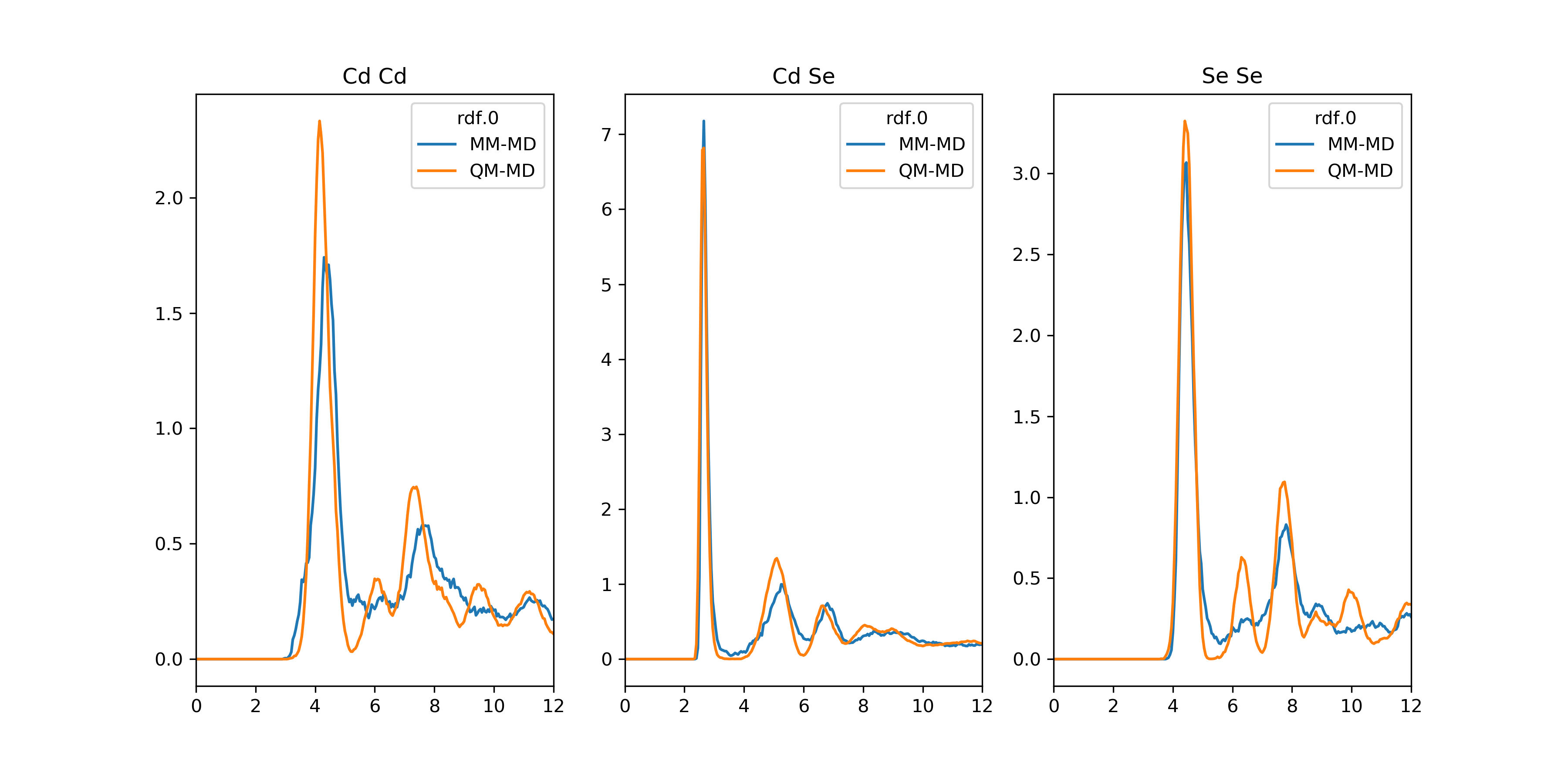Examples

A small workflow for calculating for calculating free energies using distribution functions such as the radial distribution function (RDF).

>>> import pandas as pd
>>> from FOX import get_free_energy
>>> from FOX.recipes import get_best, overlay_descriptor, plot_descriptor

>>> hdf5_file: str = ...

>>> rdf: pd.DataFrame = get_best(hdf5_file, name='rdf')
>>> G: pd.DataFrame = get_free_energy(rdf, unit='kcal/mol')

>>> rdf_dict = overlay_descriptor(hdf5_file, name='rdf)
>>> G_dict = {key: get_free_energy(value) for key, value in rdf_dict.items()}
>>> plot_descriptor(G_dict)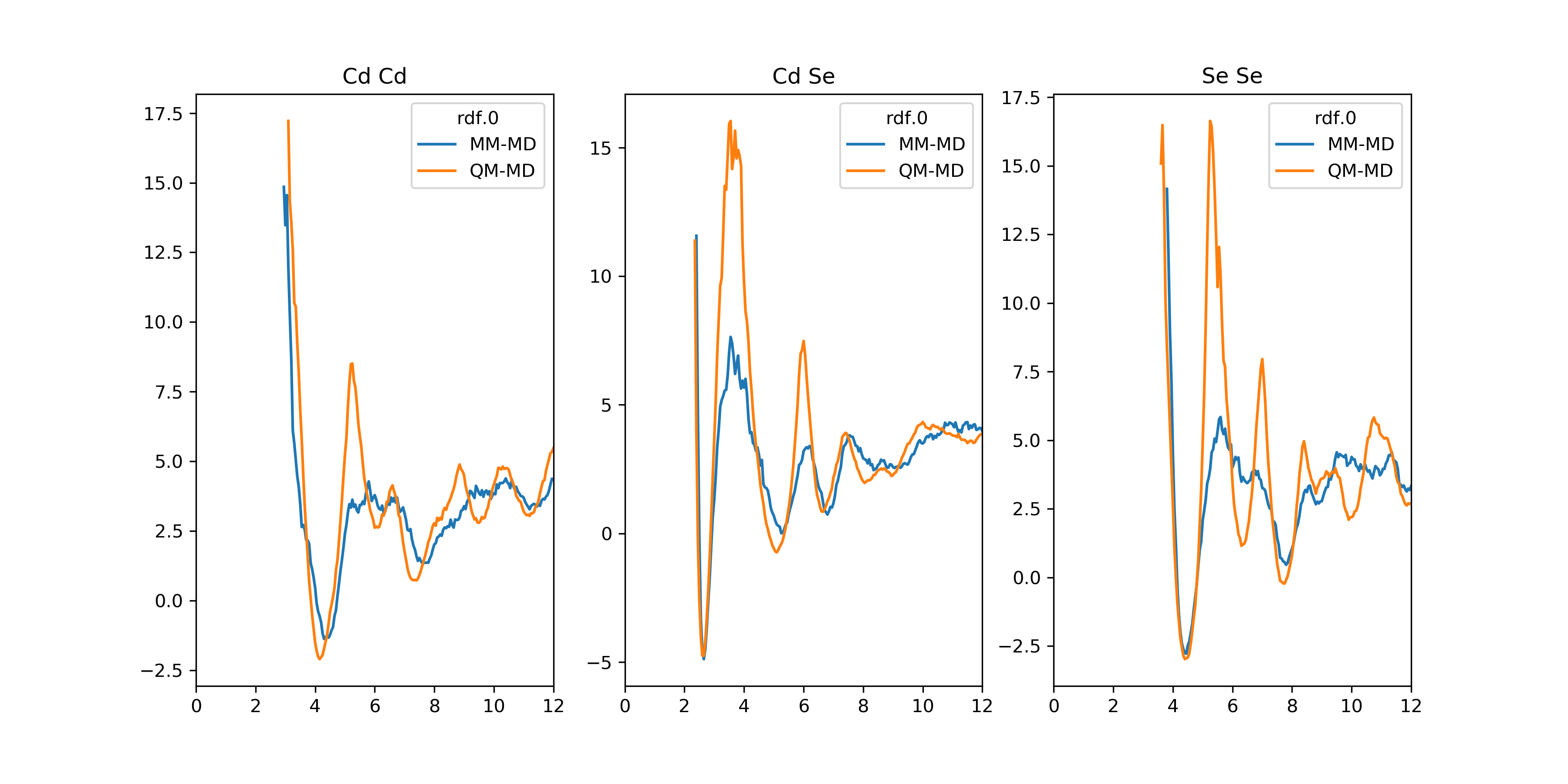Examples

A workflow for plotting parameters as a function of ARMC iterations.

>>> import numpy as np
>>> import pandas as pd
>>> from FOX import from_hdf5
>>> from FOX.recipes import plot_descriptor

>>> hdf5_file: str = ...

>>> param: pd.DataFrame = from_hdf5(hdf5_file, 'param')
>>> param.index.name = 'ARMC iteration'
>>> param_dict = {key: param[key] for key in param.columns.levels}

>>> plot_descriptor(param_dict)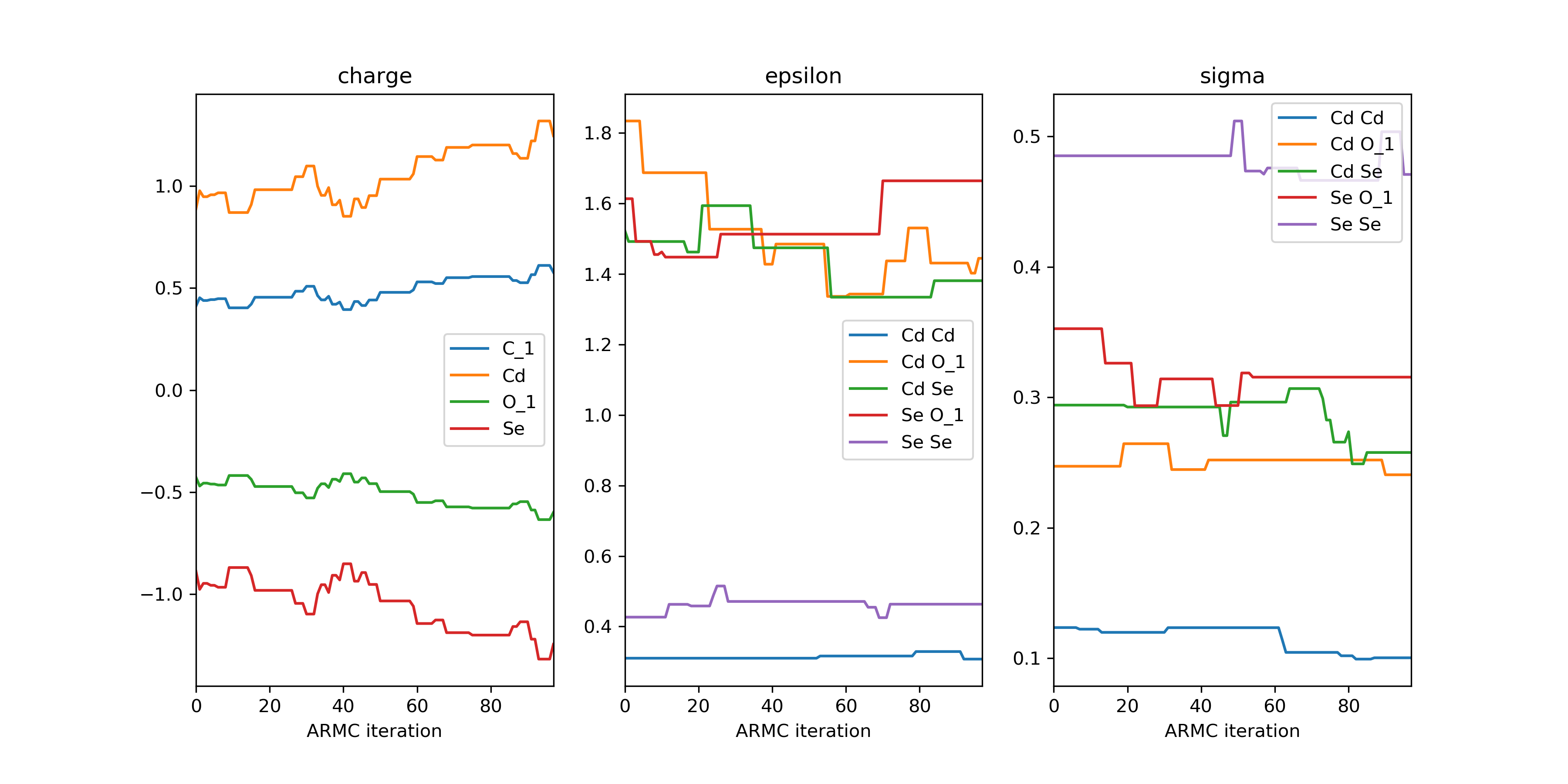This approach can also be used for the plotting of other properties such as the auxiliary error.

>>> ...

>>> err: pd.DataFrame = from_hdf5(hdf5_file, 'aux_error')
>>> err.index.name = 'ARMC iteration'
>>> err_dict = {'Auxiliary Error': err}

>>> plot_descriptor(err_dict)On occasion it might be desirable to only print the error of, for example, accepted iterations. Given a sequence of booleans (bool_seq), one can slice a DataFrame or Series (df) using df.loc[bool_seq].

>>> ...

>>> acceptance: np.ndarray = from_hdf5(hdf5_file, 'acceptance')  # Boolean array
>>> err_slice_dict = {key: df.loc[acceptance], value for key, df in err_dict.items()}

>>> plot_descriptor(err_slice_dict)


### Index

 get_best(hdf5_file, name[, i, sum_error, ...]) Return the PES descriptor or ARMC property which yields the lowest error. overlay_descriptor(hdf5_file[, name, i, ...]) Return the PES descriptor which yields the lowest error and overlay it with the reference PES descriptor. plot_descriptor(descriptor[, show_fig, ...]) Plot a DataFrame or iterable consisting of one or more DataFrames.

### API

FOX.recipes.get_best(hdf5_file, name, i=0, sum_error=None, err_dset='aux_error')[source]

Return the PES descriptor or ARMC property which yields the lowest error.

Parameters
• hdf5_file (str) – The path+filename of the ARMC .hdf5 file.

• name (str) – The name of the PES descriptor, e.g. "rdf". Alternatively one can supply an ARMC property such as "acceptance", "param" or "aux_error".

• i (int) – The index of the desired PES. Only relevant for PES-descriptors of state-averaged ARMCs.

• sum_error (str or list[str], optional) – Sum all the given aux errors for a given iteration when determining an optimum. If None, sum over all aux errors.

• err_dset (str) – The name of the dataset containing the errors. Generally speaking one should pick either "aux_error" or "validation/aux_error".

Returns

A DataFrame of the optimal PES descriptor or other (user-specified) ARMC property.

Return type

pandas.DataFrame or pd.Series

FOX.recipes.overlay_descriptor(hdf5_file, name='rdf', i=0, err_dset='aux_error')[source]

Return the PES descriptor which yields the lowest error and overlay it with the reference PES descriptor.

Parameters
• hdf5_file (str) – The path+filename of the ARMC .hdf5 file.

• name (str) – The name of the PES descriptor, e.g. "rdf".

• i (int) – The index of desired PES. Only relevant for state-averaged ARMCs.

• err_dset (str) – The name of the dataset containing the errors. Generally speaking one should pick either "aux_error" or "validation/aux_error".

Returns

A dictionary of DataFrames. Values consist of DataFrames with two keys: "MM-MD" and "QM-MD". Atom pairs, such as "Cd Cd", are used as keys.

Return type
FOX.recipes.plot_descriptor(descriptor, show_fig=True, kind='line', sharex=True, sharey=False, **kwargs)[source]

Plot a DataFrame or iterable consisting of one or more DataFrames.

Requires the matplotlib package.

Parameters
Returns

A matplotlib Figure.

Return type

Figure

get_best()

Return the PES descriptor or ARMC property which yields the lowest error.

overlay_descriptor()

Return the PES descriptor which yields the lowest error and overlay it with the reference PES descriptor.

## FOX.recipes.psf

A set of functions for creating .psf files.

Examples

Example code for generating a .psf file. Ligand atoms within the ligand .xyz file and the qd .xyz file should be in the exact same order. For example, implicit hydrogen atoms added by the from_smiles() functions are not guaranteed to be ordered, even when using canonical SMILES strings.

>>> from scm.plams import Molecule, from_smiles
>>> from FOX import PSFContainer
>>> from FOX.recipes import generate_psf

# Accepts .xyz, .pdb, .mol or .mol2 files
>>> qd = Molecule(...)
>>> ligand: Molecule = Molecule(...)
>>> rtf_file : str = ...
>>> psf_file : str = ...

>>> psf: PSFContainer = generate_psf(qd_xyz, ligand_xyz, rtf_file=rtf_file)
>>> psf.write(psf_file)


Examples

If no ligand .xyz is on hand, or its atoms are in the wrong order, it is possible the extract the ligand directly from the quantum dot. This is demonstrated below with oleate ($$C_{18} H_{33} O_{2}^{-}$$).

>>> from scm.plams import Molecule
>>> from FOX import PSFContainer
>>> from FOX.recipes import generate_psf, extract_ligand

>>> qd = Molecule(...)  # Accepts an .xyz, .pdb, .mol or .mol2 file
>>> rtf_file : str = ...

>>> ligand_len = 18 + 33 + 2
>>> ligand_atoms = {'C', 'H', 'O'}
>>> ligand: Molecule = extract_ligand(qd, ligand_len, ligand_atoms)

>>> psf: PSFContainer = generate_psf(qd, ligand, rtf_file=rtf_file)
>>> psf.write(...)


Examples

Example for multiple ligands.

>>> from typing import List
>>> from scm.plams import Molecule
>>> from FOX import PSFContainer
>>> from FOX.recipes import generate_psf2

>>> qd = Molecule(...)  # Accepts an .xyz, .pdb, .mol or .mol2 file
>>> ligands = ('C[O-]', 'CC[O-]', 'CCC[O-]')
>>> rtf_files = (..., ..., ...)

>>> psf: PSFContainer = generate_psf2(qd, *ligands, rtf_file=rtf_files)
>>> psf.write(...)


If the the psf construction with generate_psf2() failes to identify a particular ligand, it is possible to return all (failed) potential ligands with the ret_failed_lig parameter.

>>> ...

>>> ligands = ('CCCCCCCCC[O-]', 'CCCCBr')
>>> failed_mol_list: List[Molecule] = generate_psf2(qd, *ligands, ret_failed_lig=True)


### Index

 generate_psf(qd[, ligand, rtf_file, str_file]) Generate a PSFContainer instance for qd. generate_psf2(qd, *ligands[, rtf_file, ...]) Generate a PSFContainer instance for qd with multiple different ligands. extract_ligand(qd, ligand_len, ligand_atoms) Extract a single ligand from qd.

### API

FOX.recipes.generate_psf(qd, ligand=None, rtf_file=None, str_file=None)[source]

Generate a PSFContainer instance for qd.

Parameters
• qd (str or Molecule) – The ligand-pacifated quantum dot. Should be supplied as either a Molecule or .xyz file.

• ligand (str or Molecule, optional) – A single ligand. Should be supplied as either a Molecule or .xyz file.

• rtf_file (str, optional) – The path+filename of the ligand’s .rtf file. Used for assigning atom types. Alternativelly, one can supply a .str file with the str_file argument.

• str_file (str, optional) – The path+filename of the ligand’s .str file. Used for assigning atom types. Alternativelly, one can supply a .rtf file with the rtf_file argument.

Returns

A PSFContainer instance with the new .psf file.

Return type

PSFContainer

FOX.recipes.generate_psf2(qd, *ligands, rtf_file=None, str_file=None, ret_failed_lig=False)[source]

Generate a PSFContainer instance for qd with multiple different ligands.

Note

Requires the optional RDKit package.

Parameters
• qd (str or Molecule) – The ligand-pacifated quantum dot. Should be supplied as either a Molecule or .xyz file.

• *ligands (str, Molecule or Chem.Mol) – One or more PLAMS/RDkit Molecules and/or SMILES strings representing ligands.

• rtf_file (str or Iterable [str], optional) – The path+filename of the ligand’s .rtf files. Filenames should be supplied in the same order as ligands. Used for assigning atom types. Alternativelly, one can supply a .str file with the str_file argument.

• str_file (str or Iterable [str], optional) – The path+filename of the ligand’s .str files. Filenames should be supplied in the same order as ligands. Used for assigning atom types. Alternativelly, one can supply a .rtf file with the rtf_file argument.

• ret_failed_lig (bool) – If True, return a list of all failed (potential) ligands if the function cannot identify any ligands within a certain range. Usefull for debugging. If False, raise a MoleculeError.

Returns

A single ligand Molecule.

Return type

Molecule

Raises

MoleculeError – Raised if the function fails to identify any ligands within a certain range. If ret_failed_lig = True, return a list of failed (potential) ligands instead and issue a warning.

FOX.recipes.extract_ligand(qd, ligand_len, ligand_atoms)[source]

Extract a single ligand from qd.

Parameters
Returns

A single ligand Molecule.

Return type

Molecule

## FOX.recipes.ligands

A set of functions for analyzing ligands.

Examples

An example for generating a ligand center of mass RDF.

>>> import numpy as np
>>> import pandas as pd
>>> from FOX import MultiMolecule, example_xyz
>>> from FOX.recipes import get_lig_center

>>> mol = MultiMolecule.from_xyz(example_xyz)
>>> start = 123  # Start of the ligands
>>> step = 4  # Size of the ligands

# Add dummy atoms to the ligand-center of mass and calculate the RDF
>>> lig_centra: np.ndarray = get_lig_center(mol, start, step)
>>> mol_new: MultiMolecule = mol.add_atoms(lig_centra, symbols='Xx')
>>> rdf: pd.DataFrame = mol_new.init_rdf(atom_subset=['Xx'])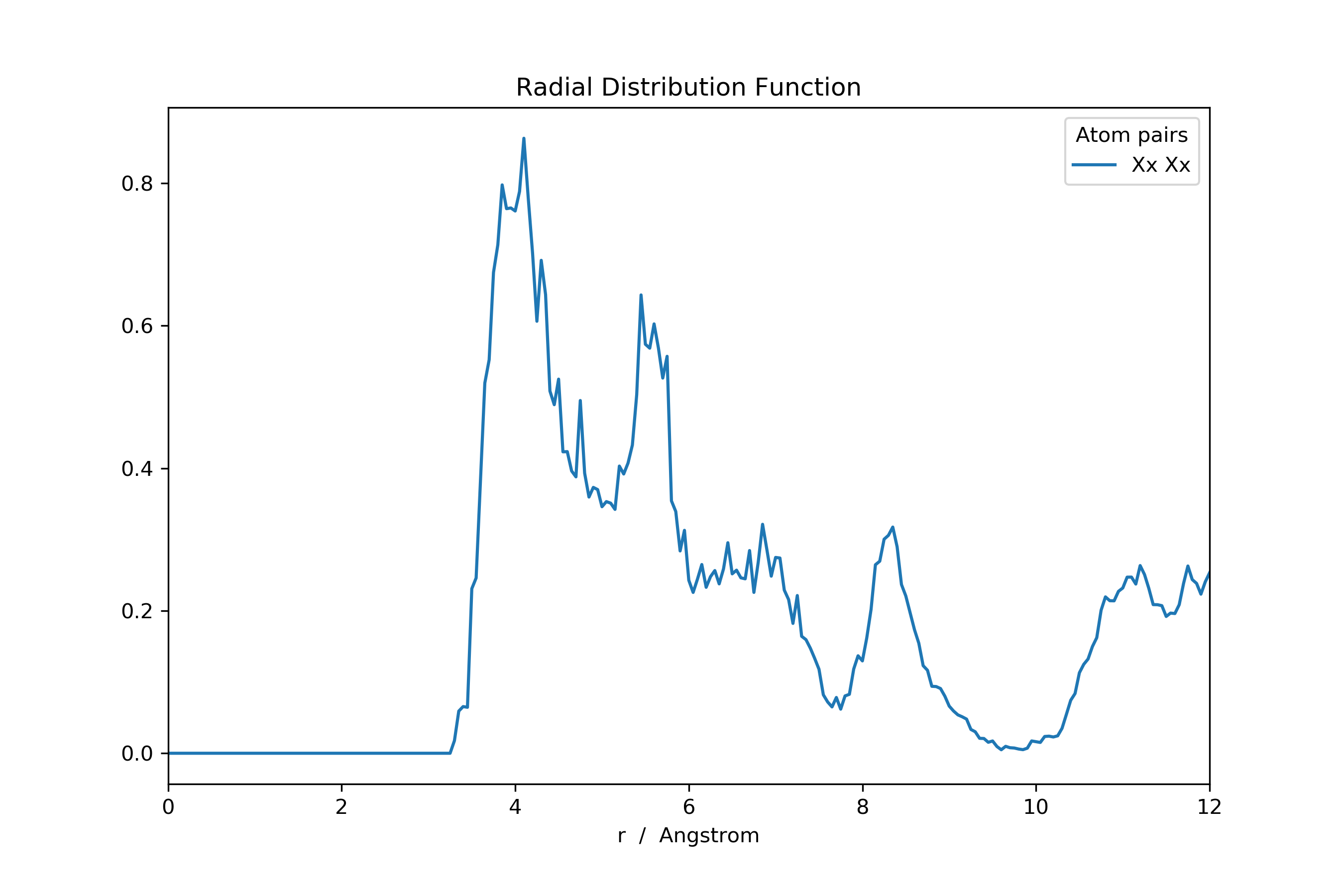>>> ...

>>> adf: pd.DataFrame = mol_new.init_rdf(atom_subset=['Xx'], r_max=np.inf)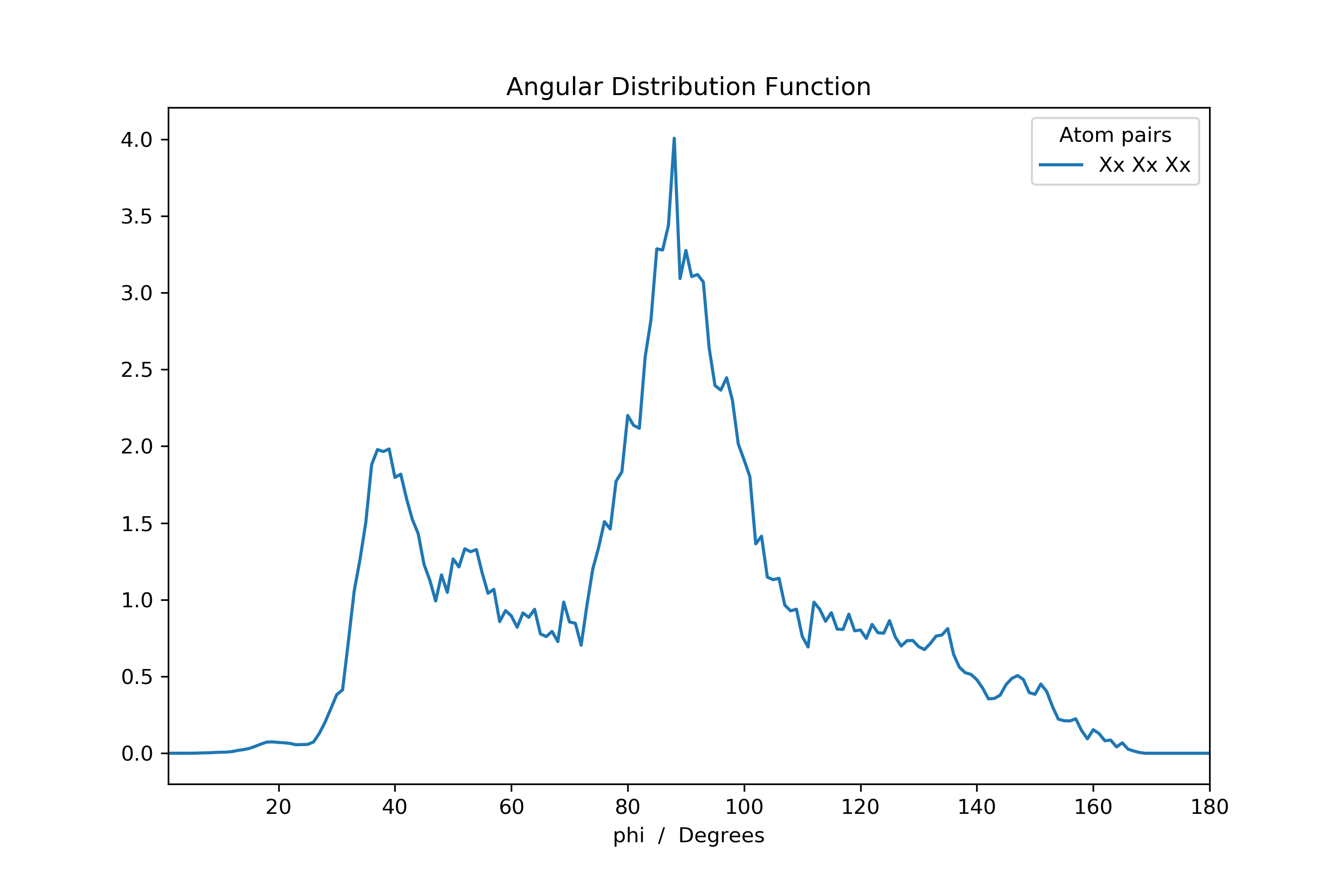Or the potential of mean force (i.e. Boltzmann-inverted RDF).

>>> ...

>>> from scipy import constants
>>> from scm.plams import Units

>>> RT: float = 298.15 * constants.Boltzmann
>>> kj_to_kcal: float = Units.conversion_ratio('kj/mol', 'kcal/mol')

>>> with np.errstate(divide='ignore'):
>>>     rdf_invert: pd.DataFrame = -RT * np.log(rdf) * kj_to_kcal
>>>     rdf_invert[rdf_invert == np.inf] = np.nan  # Set all infinities to not-a-number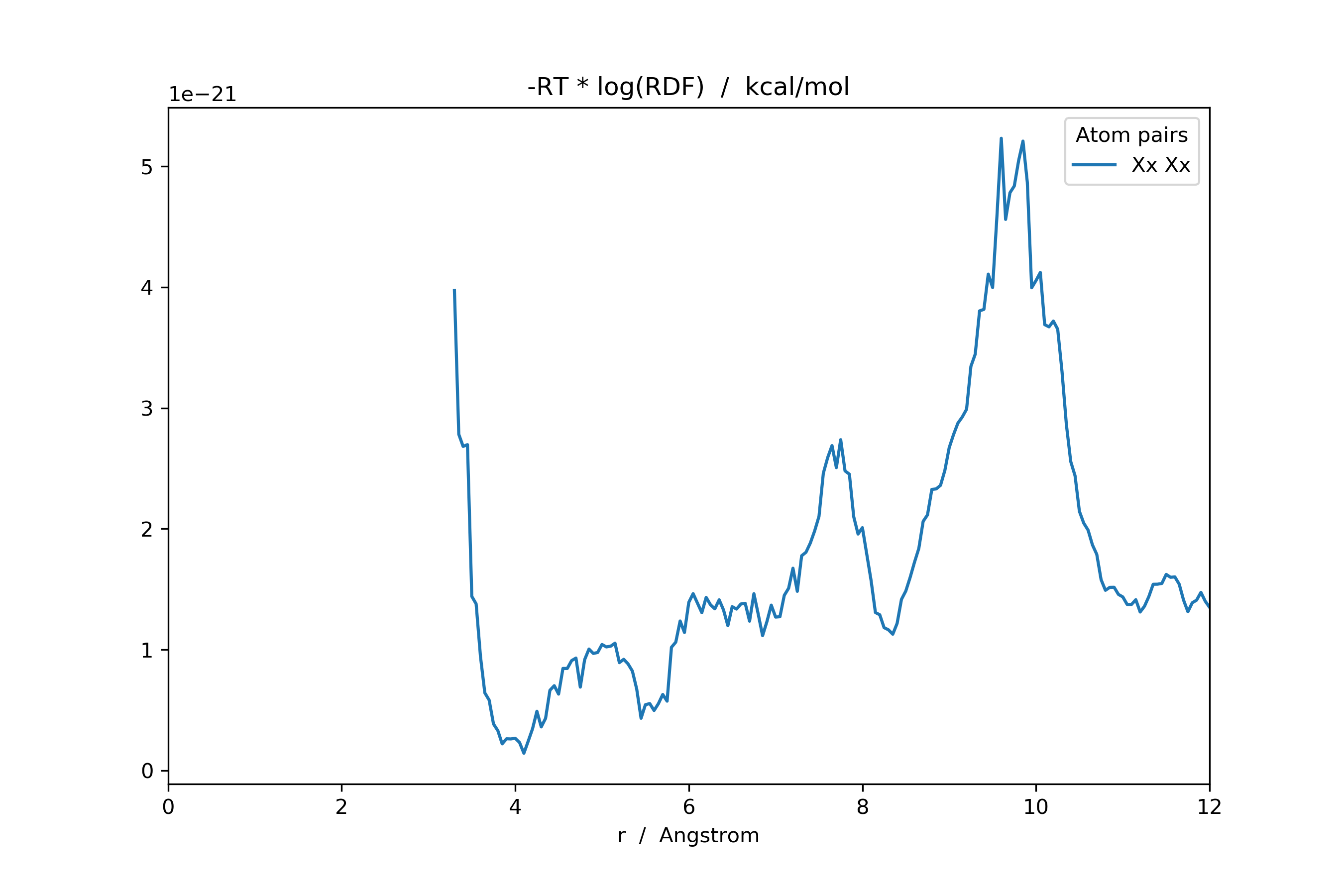Focus on a specific ligand subset is possible by slicing the new ligand Cartesian coordinate array.

>>> ...

>>> keep_lig = [0, 1, 2, 3]  # Keep these ligands; disgard the rest
>>> lig_centra_subset = lig_centra[:, keep_lig]

# Add dummy atoms to the ligand-center of mass and calculate the RDF
>>> mol_new2: MultiMolecule = mol.add_atoms(lig_centra_subset, symbols='Xx')
>>> rdf: pd.DataFrame = mol_new2.init_rdf(atom_subset=['Xx'])Examples

An example for generating a ligand center of mass RDF from a quantum dot with multiple unique ligands. A .psf file will herein be used as starting point.

>>> import numpy as np
>>> from FOX import PSFContainer, MultiMolecule, group_by_values
>>> from FOX.recipes import get_multi_lig_center

>>> mol = MultiMolecule.from_xyz(...)
>>> psf = PSFContainer.read(...)

# Gather the indices of each ligand
>>> idx_dict: dict = group_by_values(enumerate(psf.residue_id, start=1))
>>> del idx_dict  # Delete the core

# Use the .psf segment names as symbols
>>> symbols = [psf.segment_name[i].iloc for i in idx_dict.values()]

# Add dummy atoms to the ligand-center of mass and calculate the RDF
>>> lig_centra: np.ndarray = get_multi_lig_center(mol, idx_dict.values())
>>> mol_new: MultiMolecule = mol.add_atoms(lig_centra, symbols=symbols)
>>> rdf = mol_new.init_rdf(atom_subset=set(symbols))


### Index

 get_lig_center(mol, start, step[, stop, ...]) Return an array with the (mass-weighted) mean position of each ligands in mol. get_multi_lig_center(mol, idx_iter[, ...]) Return an array with the (mass-weighted) mean position of each ligands in mol.

### API

FOX.recipes.get_lig_center(mol, start, step, stop=None, mass_weighted=True)[source]

Return an array with the (mass-weighted) mean position of each ligands in mol.

Parameters
Returns

A new array with the ligand’s centra of mass. If mol.shape == (m, n, 3) then, given k new ligands, the to-be returned array’s shape is (m, k, 3).

Return type

numpy.ndarray

FOX.recipes.get_multi_lig_center(mol, idx_iter, mass_weighted=True)[source]

Return an array with the (mass-weighted) mean position of each ligands in mol.

Contrary to get_lig_center(), this function can handle molecules with multiple non-unique ligands.

Parameters
Returns

A new array with the ligand’s centra of mass. If mol.shape == (m, n, 3) then, given k new ligands (aka the length of idx_iter) , the to-be returned array’s shape is (m, k, 3).

Return type

numpy.ndarray

## FOX.recipes.time_resolution

A set of functions for calculating time-resolved distribution functions.

### Index

 time_resolved_rdf(mol[, start, stop, step]) Calculate the time-resolved radial distribution function (RDF). time_resolved_adf(mol[, start, stop, step]) Calculate the time-resolved angular distribution function (ADF).

### API

FOX.recipes.time_resolved_rdf(mol, start=0, stop=None, step=500, **kwargs)[source]

Calculate the time-resolved radial distribution function (RDF).

Examples

>>> from FOX import MultiMolecule, example_xyz
>>> from FOX.recipes import time_resolved_rdf

# Calculate each RDF over the course of 500 frames
>>> time_step = 500
>>> mol = MultiMolecule.from_xyz(example_xyz)

>>> rdf_list = time_resolved_rdf(
...     mol, step=time_step, atom_subset=['Cd', 'Se']
... )

Parameters
Returns

A list of dataframes, each containing an RDF calculated over the course of step frames.

Return type

List[pandas.DataFrame]

init_rdf()

Calculate the radial distribution function.

FOX.recipes.time_resolved_adf(mol, start=0, stop=None, step=500, **kwargs)[source]

Calculate the time-resolved angular distribution function (ADF).

Examples

>>> from FOX import MultiMolecule, example_xyz
>>> from FOX.recipes import time_resolved_adf

# Calculate each ADF over the course of 500 frames
>>> time_step = 500
>>> mol = MultiMolecule.from_xyz(example_xyz)

>>> rdf_list = time_resolved_adf(
...     mol, step=time_step, atom_subset=['Cd', 'Se']
... )

Parameters
Returns

A list of dataframes, each containing an ADF calculated over the course of step frames.

Return type

List[pandas.DataFrame]

init_adf()

Calculate the angular distribution function.

## FOX.recipes.similarity

Recipes for computing the similarity between trajectories.

Examples

An example where, starting from two .xyz files, the similarity is computed between two molecular dynamics (MD) trajectories.

>>> import numpy as np
>>> import FOX
>>> from FOX.recipes import compare_trajectories

# The relevant multi-xyz files
>>> md_filename: str = ...
>>> md = FOX.MultiMolecule.from_xyz(md_filename)
>>> md_ref_filename: str = ...
>>> md_ref = FOX.MultiMolecule.from_xyz(md_ref_filename)

# Calculate the similarity between md and md_ref
>>> similarity = compare_trajectories(md, md_ref, metric="cosine")

# Identify all sufficiently dissimilar molecules (as defined via threshold)
>>> threshold: float = ...
>>> idx = np.zeros(len(md), dtype=np.bool_)
>>> idx[similarity >= threshold] = True


The resulting indices can be used for, for example, identifying all molecules one wants to use for further (quantum-mechanical/classical) calculations.

>>> import qmflows

# Define the job settings
>>> s = qmflows.Settings()
>>> s.lattice = [50, 50, 50]
>>> s.specific.cp2k.motion.print["forces on"].filename = ""
>>> s.overlay(qmflows.templates.singlepoint)

# Construct the job list
>>> mol_list = md[idx].as_Molecule()
>>> job_list = [qmflows.cp2k(s, mol) for mol in mol_list]

# Run the jobs
>>> result_list = [qmflows.run(job) for job in job_list]

# Extract the forces and energies from all jobs
>>> forces = np.array([r.forces for r in result_list])[:, 0]
>>> energy = np.array([r.energy for r in result_list])[:, 0]


### Index

 compare_trajectories(md, md_ref, *[, ...]) Compute the similarity between 2 trajectories according to the specified metric. fps_reduce(dist_mat[, n]) Return the indices that yield a uniform distribution of n points.

### API

FOX.recipes.compare_trajectories(md, md_ref, *, metric='cosine', reduce=<function mean>, reset_origin=True, **kwargs)[source]

Compute the similarity between 2 trajectories according to the specified metric.

The default metric aliases scipy.spatial.distance.cdist() for defining the (dis-)similarity between the passed md and its reference. This (dis-)similarity array is subsequently reduced to a vector of size $$(N_{mol},)$$ by taking its mean (along the relevant axes).

Examples

>>> import numpy as np
>>> from FOX.recipes import compare_trajectories

>>> md: np.ndarray = ...
>>> md_ref: np.ndarray = ...

# Default metric presets
>>> metric1 = compare_trajectories(md, md_ref, metric="cosine")
>>> metric2 = compare_trajectories(md, md_ref, metric="euclidean")
>>> metric3 = compare_trajectories(md, md_ref, metric="minkowski", p=1)

>>> def rmsd(a: np.ndarray) -> np.float64:
...     '''Calculate the root-mean-square deviation.'''
...     return np.mean(a**2)**0.5

# Sum over the number of atoms rather than average
>>> metric4 = compare_trajectories(md, md_ref, reduce=np.sum)
>>> metric5 = compare_trajectories(md, md_ref, reduce=rmsd)

>>> def sqeuclidean(md: np.ndarray, md_ref: np.ndarray) -> np.ndarray:
...     '''Calculate the distance based on the squared eclidian norm.'''
...     delta = md[..., None] - md_ref[..., None, :]
...     return np.linalg.norm(delta, axis=-1)**2

# Pass a custom metric-function
>>> metric6 = compare_trajectories(md, md_ref, metric=sqeuclidean)

Parameters
• md (array_like, shape $$(N_{mol}, N_{atom1}, 3)$$ or $$(N_{atom1}, 3)$$) – An array-like object containing the trajectory of interest.

• md_ref (array_like, shape $$(N_{mol}, N_{atom2}, 3)$$ or $$(N_{atom2}, 3)$$) – An array-like object containing the reference trajectory.

• metric (str or Callable[[FOX.MultiMolecule, FOX.MultiMolecule], np.ndarray]) – The type of metric used for calculating the (dis-)similarity. Accepts either a callback or predefined alias. See metric parameter in scipy.spatial.distance.cdist() for a comprehensive overview of all aliases. If a callback is provided then it should take a array of shape $$(n_{atom1}, 3)$$ and $$(N_{atom2}, 3)$$ as arguments and return a new array of shape $$(N_{atom1}, N_{atom2})$$.

• reduce (Callable[[np.ndarray], np.number], optional) – A callable for performing a dimensional reduction. Used for transforming the shape $$(N_{atom1}, N_{atom2})$$ array, returned by metric, into a scalar. Setting this value to None will disable the reduction and return the metric output in unaltered form.

• reset_origin (bool) – Reset the origin by removing translations and rotations from the passed trajectories.

• **kwargs (Any) – Further keyword arguments for metric.

Returns

An array with the (dis-)similarity between all molecules in md and md_ref.

Return type

np.ndarray[np.float64], shape $$(N_{mol},)$$

scipy.spatial.distance.cdist()

Compute distance between each pair of the two collections of inputs.

FOX.recipes.fps_reduce(dist_mat, n=1, **kwargs)[source]

Return the indices that yield a uniform distribution of n points.

Examples

>>> from functools import partial
>>> import numpy as np
>>> from FOX.recipes import compare_trajectories, fps_reduce

>>> md: np.ndarray = ...
>>> md_ref: np.ndarray = ...

>>> reduce_func = partial(fps_reduce, n=10)
>>> out = compare_trajectories(md, md_ref, reduce=reduce_func)


Note

This function requires the Compound Attachment Tools package: CAT.

Parameters
Returns

An array of indices.

Return type

np.ndarray[np.int64], shape $$(n,)$$

CAT.distribution.uniform_idx()
FOX.recipes.compare_trajectories()